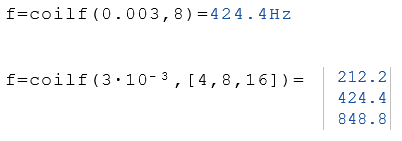# Coilf Function

Calculates the frequency for the specified coil and impedance

## Calculate the frequency

The Coilf function calculates the frequency for the specified coil and impedance. The parameters for the coil L and the impedance Z can be real numbers or lists of real numbers.

Coilf (L, Z)

### Legend

 L Inductance in Henry Z Impedance in Ohm

### Example